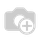# Add Any Number To Date##### 2 答案

Hi,

Write and onchange function for the integer field and using the time Delta you can add this integer to the date.

Suppose if you have a date field date_1 then in on change of the integer field field_1 add the integer to date by timedelta.

In on change of field_1,

New_date = date_1 +timedelta(days=self.field_1)

ThanksHi,

You can write as following:

```@api.onchange('integer_field')
def onchange_next_order_date(self):
if self.date_field :
next_order_d1_obj = datetime.datetime.strptime(self.date_field, "%Y-%m-%d")
next_date = next_order_d1_obj + datetime.timedelta(days=self.integer_field)
self.date_field  = next_date.strftime("%Y-%m-%d")```

Thanks